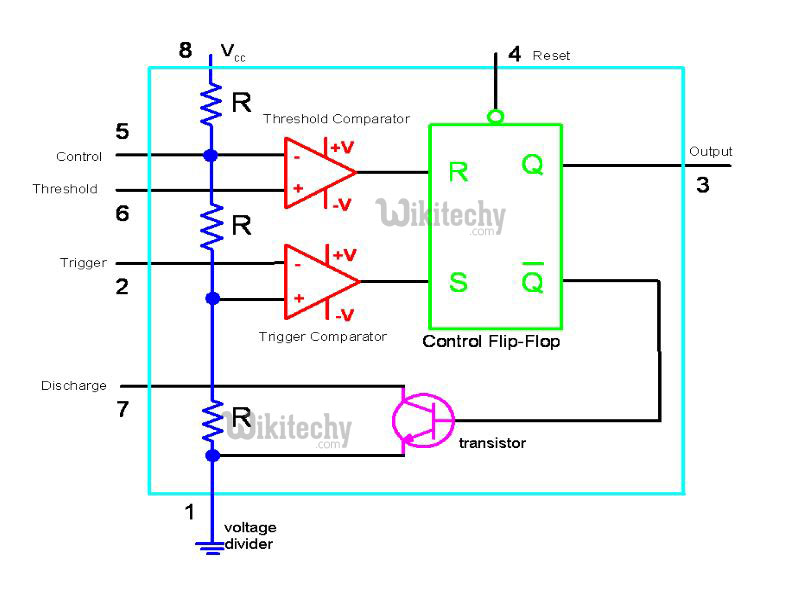# 555 Timer LED Flasher - Block Diagram of IC 555 Timer

## Block DiagramBlock Diagram of IC 555

## Inside the 555 Timer

• The voltage divider (blue) has three equal 5K resistors. It divides the input voltage (Vcc) into three equal parts.
• The two comparators (red) are op-amps that compare the voltages at their inputs and saturate depending upon which is greater.
• Threshold Comparator
• Trigger Comparator
• The Threshold Comparator saturates when the voltage at the Threshold pin (pin 6) is greater than (2/3)Vcc.
• The Trigger Comparator saturates when the voltage at the Trigger pin (pin 2) is less than (1/3)Vcc
• The flip-flop (green) is a bi-stable device. It generates two values, a “high” value equal to Vcc and a “low” value equal to 0V.
• When the Threshold comparator saturates, the flip flop is Reset (R) and it outputs a low signal at pin 3.
• When the Trigger comparator saturates, the flip flop is Set (S) and it outputs a high signal at pin 3.
• The transistor (purple) is being used as a switch, it connects pin 7 (discharge) to ground when it is closed.
• When Q is low, Qbar is high. This closes the transistor switch and attaches pin 7 to ground.
• When Q is high, Qbar is low. This open the switch and pin 7 is no longer grounded